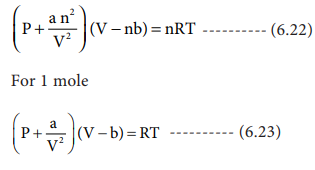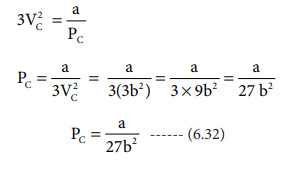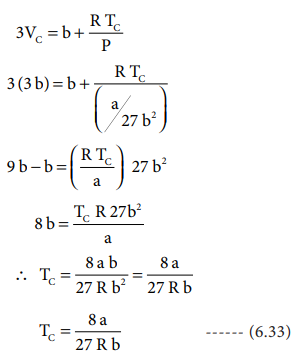Home | | Chemistry 11th std | Derivation of critical constants from van der Waals constant

# Derivation of critical constants from van der Waals constant

From the equation we can derive the values of critical constants Pc, Vc and Tc in terms of a and b, the van der Waals constants.

Derivation of critical constants from van der Waals constant:

The van der Waals equation for n moles isFrom the equation we can derive the values of critical constants Pc, Vc and Tc in terms of a and b, the van der Waals constants, On expanding the above equationWhen the above equation is rearranged in powers of VThe equation (6.26) is a cubic equation in V. On solving this equation, we will get three solutions. At the critical point all these three solutions of V are equal to the critical volume VC. The pressure and temperature becomes Pc and Tc respectively

V = VC

V â€“ VC = 0

(V â€“ VC)3 = 0

V3 - 3VCV3 + 3VC2V - VC3 = 0 .......(6.27)

As equation (6.26) is identical with equation (6.27), we can equate the coefficients of V2, V and constant terms in (6.26) and (6.27).i.e. VC = 3b -------   (6.31)

when equation (6.31) is substituted in (6.29)substituting the values of Vc and Pc in equation (6.28),The critical constants can be calculated using the values of van der waals constant of a gas and vice versa.Study Material, Lecturing Notes, Assignment, Reference, Wiki description explanation, brief detail
11th Chemistry : UNIT 6 : Gaseous State : Derivation of critical constants from van der Waals constant |